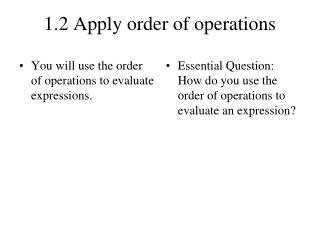DownloadDownload Presentation1.2 Apply order of operations

# 1.2 Apply order of operations

Download Presentation## 1.2 Apply order of operations

- - - - - - - - - - - - - - - - - - - - - - - - - - - E N D - - - - - - - - - - - - - - - - - - - - - - - - - - -
##### Presentation Transcript

1. 1.2 Apply order of operations • You will use the order of operations to evaluate expressions. • Essential Question: How do you use the order of operations to evaluate an expression?

2. Evaluate the expression27 32 2 3. 27 32 2 – 3 = 27 92 – 3 32–3=6–3 279 2 – 3 =3 2 – 3 EXAMPLE 1 Evaluate expressions – STEP 1 There are no grouping symbols, so go to Step 2. STEP 2 Evaluatepowers. Evaluate power. STEP 3 Multiply and divide from left to right. Divide. Multiply.

3. ANSWER The value of the expression27 32 2 – 3 is 3. EXAMPLE 1 Evaluate expressions STEP 4 Add and subtract from left to right. 6 – 3 = 3 Subtract.

4. 2. Evaluate the expression2 32 + 4 3. Evaluate the expression 32 23 + 6 ANSWER ANSWER 1. 4 2. 22 ANSWER 3.10 for Example 1 GUIDED PRACTICE 1. Evaluate the expression20 – 42

5. ANSWER 4. 47 for Example 1 GUIDED PRACTICE 4. Evaluate the expression 15 + 62– 4

6. 35 = 24 – (32+ 1) = b. = 24 – 10 14 = 2 = 18 = EXAMPLE 2 Evaluate expressions with grouping symbols Evaluate the expression. 7(5) a. 7(13 – 8) = Subtract within parentheses. Multiply. 24 – (9+ 1) Evaluate power. Add within parentheses. Subtract. 2[30 –21] c. 2[30 – (8+ 13)] = Add within parentheses. Subtract within brackets. Multiply.

7. 9 4 9x = 3(4+ 2) 3(x+ 2) 9 4 = 3 6 36 = 18 = 2 EXAMPLE 3 Evaluate an algebraic expression Evaluate the expression whenx=4. Substitute 4 for x. Add within parentheses. Multiply. Divide.

8. = 48 3(8– 22) 6. 7. 2[( 9 + 3) 4 ] for Examples 2 and 3 GUIDED PRACTICE Evaluate the expression. 5. 4(3+ 9) = 12 = 6

9. 8. 9. 12 – y – 1 y2– 3 61 = 10y + 1 10. y + 1 3 = for Examples 2 and 3 GUIDED PRACTICE Evaluate the expression wheny= 8. = 9

10. EXAMPLE 4 Standardized Test Practice

11. =12(31.25+ 22)+ 30 ANSWER The sponsor’s cost is \$123.The correct answer is B. . D C A B EXAMPLE 4 Standardized Test Practice SOLUTION 12(3j+2s) +30 Substitute 1.25forj and 2 for s. = 12(3.75 + 4) + 30 Multiply within parentheses. = 12(7.75) + 30 Add within parentheses. = 93 + 30 Multiply. = 123 Add.

12. ANSWER The sponsor’s cost is \$216.No; the total cost of the juice drinks and sandwiches will double, but the cost of the trash bags will not. for Example 4 GUIDED PRACTICE 11. WHAT IF? In Example 4, suppose the number of volunteers doubles. Does the sponsor’s cost double as well? Explain.

13. 1. 33  9 + 4 3 15 ANSWER ANSWER 16 ANSWER 40 Daily Homework Quiz Evaluate the expression. 2. 12 + (5 – 3)2 3.2(c2 + 4 ) whenc = 4

14. 4. when g = 6 4 ANSWER • The cost (in dollars) to buy p posters at the Posters Plus online store is given by the expression 3.5p + 6.75. At the More Posters online store, the cost is given by 4.25p +2.55. Which store has the lower cost for 5 posters? 5. g2 – 8 2g – 5 ANSWER The cost at the More Posters online store is less. Daily Homework Quiz

15. You will use the order of operations to evaluate expressions. • Essential Question: How do you use the order of operations to evaluate an expression? The order of operations is a series of steps to use when evaluating expressions. Parentheses, brackets, and fraction bars act as grouping symbols. Always evaluate expressions using the following order: evaluate expressions inside grouping symbols, evaluate powers, multiply and divide from left to right, and then add and subtract from left to right.